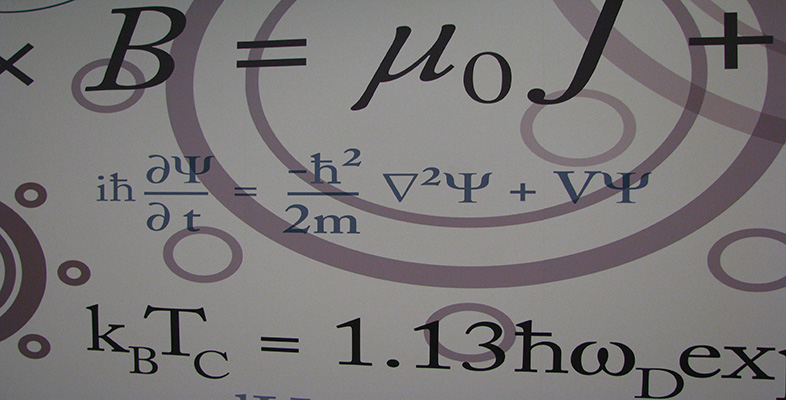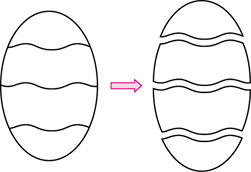Number systems

This free course is available to start right now. Review the full course description and key learning outcomes and create an account and enrol if you want a free statement of participation.

Free course

4 Equivalence relations

4.1 What is a relation?

In this final section we look at a method of classifying the elements of a set by sorting them into subsets. We shall require that the set is sorted into disjoint subsets – so each element of the set belongs to exactly one subset. Such a classification is known as a partition of a set. In order to achieve a partition, we need to have a method which enables us to decide whether or not one element belongs to the same subset as another. We look first at the general idea of a relation, and then at the particular properties needed by a relation in order to partition a set. A relation which satisfies these special properties is known as an equivalence relation, and the subsets into which the set is partitioned are called equivalence classes.Equivalence relations occur in all branches of mathematics. For example, in geometry, two possible relations between the set of all triangles in the plane are is congruent to and is similar to. These are both equivalence relations: the relation is congruent to partitions the set of triangles into classes such that all the triangles within each class are congruent to each other, whereas is similar to partitions the set into classes of similar triangles. These partitions are different: triangles of the same shape but different sizes are similar to, but not congruent to, each other.

Equivalence relations are not confined to sets of mathematical objects. For example, relations between people such as is the same height as and has the same birthday as are equivalence relations.

M208_6